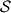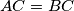IMO Shortlist 2015 problem C2

Kvaliteta:
Avg: 0,0
Težina:
Avg: 6,0

We say that a finite set$\mathcal{S}$ of points in the plane is balanced if, for any two different points$A$ and$B$ in$\mathcal{S}$, there is a point$C$ in$\mathcal{S}$ such that$AC=BC$. We say that$\mathcal{S}$ is centre-free if for any three different points$A$,$B$ and$C$ in$\mathcal{S}$, there is no points$P$ in$\mathcal{S}$ such that$PA=PB=PC$.

(a) Show that for all integers$n\ge 3$, there exists a balanced set consisting of$n$ points.

(b) Determine all integers$n\ge 3$ for which there exists a balanced centre-free set consisting of$n$ points.

(Netherlands)

Izvor: https://www.imo-official.org/problems/IMO2015SL.pdf